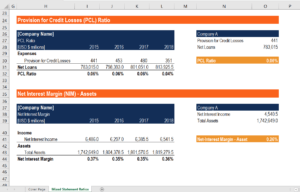# Bank Mixed Statement Ratio Calculator

## Bank Mixed Statement Ratio Calculator

The Bank Mixed Statement Ratio Calculator can be used to determine a bank’s profitability and financial strength using items found on an income statement and balance sheet.

This template includes the following ratios:

• Return on Assets (ROA) Ratio
• Return on Equity (ROE) Ratio
• Provision for Credit Losses (PCL) Ratio
• Net Interest Margin (NIM) – Assets

Here is a quick preview of CFI’s Bank Mixed Statement Ratio Calculator:### Return on Assets (ROA) Ratio

The return on assets ratio allows investors to compare the profitability of companies within the same industries. This is possible as the ratio shows the profit of a bank as a percentage of the assets. Thus, the size and amount of asset a company is taken into account.

The formula for the return on assets ratio is:

Return on Assets = Net Income / End of Period Assets

### Return on Equity (ROE) Ratio

The return on equity ratio demonstrates the ability of a company to utilize its equity to generate profits. It is similar to ROA except net income is compared to equity instead of assets. The ROE also allows investors to compare a bank’s profitability to the industry average or other companies.

The formula for the return on equity ratio is:

Return on Equity = Net Income / Shareholders’ Equity

### Provision for Credit Losses (PCL) Ratio

The provision for credit losses ratio is used to evaluate the provision as a percentage of a bank’s loan book. Provision is the amount of capital a bank sets aside to cover loans that might not be recovered. A consistently high PCL ratio suggests the bank has a tendency to underwrite risky loans.

The formula for provision for credit losses ratio is:

Provision for Credit Losses Ratio =  Provision for Credit Losses / Net Loans and Acceptances

### Net Interest Margin (NIM) – Assets

This ratio represents net interest income as a percentage of total assets. This is a profitability ratio that only accounts for interest income generated through assets. Therefore, income generated through transaction, account, and advisory fees is not included.

The formula for net interest margin is:

Net Interest Margin = (Interest Income – Interest Expense) / Total Assets